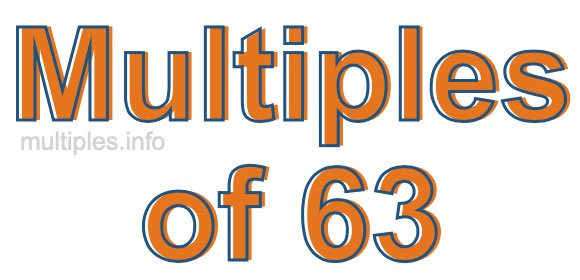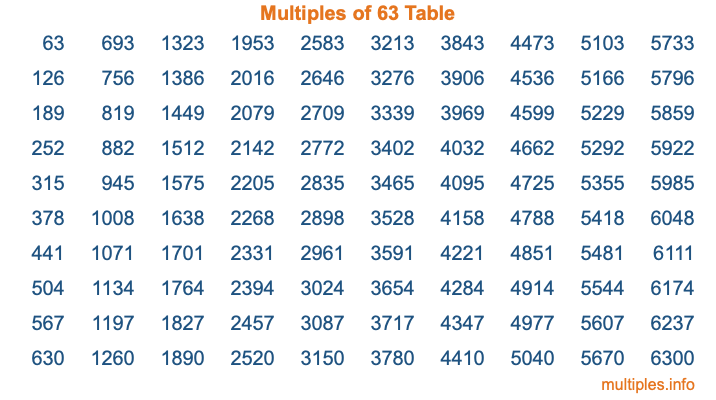Multiples of 63Welcome to the Multiples of 63 page. Here we will first teach you everything you will ever need to know about the multiples of 63, and then give you a study guide summary of everything we taught you to make sure you remember it all. Use this page to look up facts and learn information about the multiples of 63. This page will make you a multiples of sixty-three expert!

Definition of Multiples of 63
Multiples of 63 are all the numbers that when divided by 63 equal an integer. Each of the multiples of 63 are called a multiple. A multiple of 63 is created by multiplying 63 by an integer.

Therefore, to create a list of multiples of 63, you start with 1 multiplied by 63, then 2 multiplied by 63, then 3 multiplied by 63, and so on for as long as you want. Thus, the list of the first five multiples of 63 is 63, 126, 189, 252, and 315. To see a larger list of multiples of 63, see the printable image of Multiples of 63 further down on this page. We also have a category where you can choose any nth multiple of 63.

Multiples of 63 Checker
The Multiples of 63 Checker below checks to see if any number of your choice is a multiple of 63. In other words, it checks to see if there is any number (integer) that when multiplied by 63 will equal your number. To do that, we divide your number by 63. If the the quotient is an integer, then your number is a multiple of 63.

Is  a multiple of 63?

Least Common Multiple of 63 and ...
A Least Common Multiple (LCM) is the lowest multiple that two or more numbers have in common. This is also called the smallest common multiple or lowest common multiple and is useful to know when you are adding our subtracting fractions. Enter one or more numbers below (63 is already entered) to find the LCM.

Check out our LCM Calculator if you need more details about the Least Common Multiple or if you need the LCM for different numbers for adding and subtraction fractions.

nth Multiple of 63
As we stated above, 63 is the first multiple of 63, 126 is the second multiple of 63, 189 is the third multiple of 63, and so on. Enter a number below to find the nth multiple of 63.

th multiple of 63

Multiples of 63 vs Factors of 63
63 is a multiple of 63 and a factor of 63, but that is where the similarities end. All postive multiples of 63 are 63 or greater than 63. All positive factors of 63 are 63 or less than 63.

Below is the beginning list of multiples of 63 and the factors of 63 so you can compare:

Multiples of 63: 63, 126, 189, 252, 315, etc.

Factors of 63: 1, 3, 7, 9, 21, 63

As you can see, the multiples of 63 are all the numbers that you can divide by 63 to get a whole number. The factors of 63, on the other hand, are all the whole numbers that you can multiply by another whole number to get 63.

It's also interesting to note that if a number (x) is a factor of 63, then 63 will also be a multiple of that number (x).

Multiples of 63 vs Divisors of 63
The divisors of 63 are all the integers that 63 can be divided by evenly. Below is a list of the divisors of 63.

Divisors of 63: 1, 3, 7, 9, 21, 63

The interesting thing to note here is that if you take any multiple of 63 and divide it by a divisor of 63, you will see that the quotient is an integer.

Multiples of 63 Table
Below is an image of the first 100 multiples of 63 in a table. The table is in chronological order, column by column. The first column has the first ten multiples of 63, the second column has the next ten multiples of 63, and so on.The Multiples of 63 Table is also referred to as the 63 Times Table or Times Table of 63. You are welcome to print out our table for your studies.

Negative Multiples of 63
Although not often discussed or needed in math, it is worth mentioning that you can make a list of negative multiples of 63 by multiplying 63 by -1, then by -2, then by -3, and so on, to get the following list of negative multiples of 63:

-63, -126, -189, -252, -315, etc.

Multiples of 63 Summary
Below is a summary of important Multiples of 63 facts that we have discussed on this page. To retain the knowledge on this page, we recommend that you read through the summary and explain to yourself or a study partner why they hold true.

There are an infinite number of multiples of 63.

A multiple of 63 divided by 63 will equal a whole number.

63 divided by a factor of 63 equals a divisor of 63.

The nth multiple of 63 is n times 63.

The largest factor of 63 is equal to the first positive multiple of 63.

63 is a multiple of every factor of 63.

63 is a multiple of 63.

A multiple of 63 divided by a divisor of 63 equals an integer.

63 divided by a divisor of 63 equals a factor of 63.

Any integer times 63 will equal a multiple of 63.

Multiples of a Number
Here you can get the multiples of another number, all with the same attention to detail as we did for multiples of 63 on this page.

Multiples of
Multiples of 64
Did you find our page about multiples of sixty-three educational? Do you want more knowledge? Check out the multiples of the next number on our list!